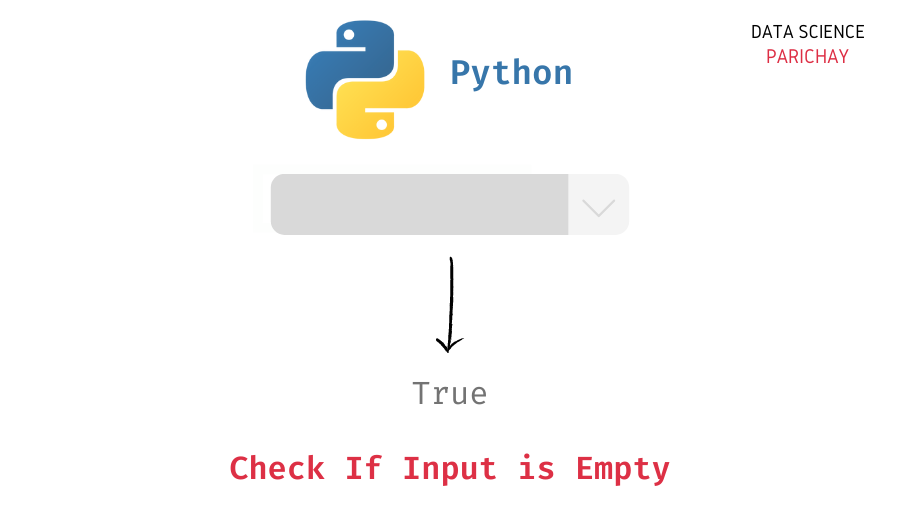# Python – Check If Input Is Empty

In this tutorial, we will look at how to check if the user input in Python is empty or not with the help of some examples.

## How to check if user input is empty?

Values from the standard input are read as strings in Python. So, to check if the input is empty use the equality operator `==` in Python to check if the input string is equal to an empty string or not.

The following is the syntax –

```# check if input is empty
s = input()
print(s == '')```

It returns `True` if the input string is empty and `False` otherwise

Let’s now look at some examples of using the above syntax –

First, let’s take an empty input using the `input()` function and then check if it’s empty or not.

```# take input from user
s = input()
# check if input is emtpy
print(s == '')```

Output:

`True`

Here, we gave an empty input. Since the input string is an empty string, we get `True` as the output.

📚 Data Science Programs By Skill Level

Introductory

Intermediate ⭐⭐⭐

🔎 Find Data Science Programs 👨‍💻 111,889 already enrolled

Disclaimer: Data Science Parichay is reader supported. When you purchase a course through a link on this site, we may earn a small commission at no additional cost to you. Earned commissions help support this website and its team of writers.

Let’s try another example. This time we’ll pass a value to the input.

```# take input from user
s = input()
# check if input is emtpy
print(s == '')```

Output:

```cat
False```

We get `False` as the output since the input string is non-empty.

Note that, if you pass one or more spaces as input, it will be considered as a non-empty string.

```# take input from user
s = input()
# check if input is emtpy
print(s == '')```

Output:

```
False```

We get `False` as the output.

In case, you want to omit the starting and trailing spaces from the entered string, you can the string `strip()` function and then check for the empty input.

```# take input from user
s = input()
# check if input is emtpy
print(s.strip() == '')```

Output:

`True`

Now, we get `True` as the output.

You might also be interested in –

•